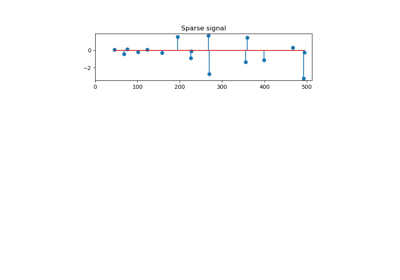/scikit-learn

# sklearn.datasets.make_sparse_coded_signal

`sklearn.datasets.make_sparse_coded_signal(n_samples, n_components, n_features, n_nonzero_coefs, random_state=None)` [source]

Generate a signal as a sparse combination of dictionary elements.

Returns a matrix Y = DX, such as D is (n_features, n_components), X is (n_components, n_samples) and each column of X has exactly n_nonzero_coefs non-zero elements.

Read more in the User Guide.

Parameters: `n_samples : int` number of samples to generate `n_components : int,` number of components in the dictionary `n_features : int` number of features of the dataset to generate `n_nonzero_coefs : int` number of active (non-zero) coefficients in each sample `random_state : int, RandomState instance or None (default)` Determines random number generation for dataset creation. Pass an int for reproducible output across multiple function calls. See Glossary. `data : array of shape [n_features, n_samples]` The encoded signal (Y). `dictionary : array of shape [n_features, n_components]` The dictionary with normalized components (D). `code : array of shape [n_components, n_samples]` The sparse code such that each column of this matrix has exactly n_nonzero_coefs non-zero items (X).

## Examples using `sklearn.datasets.make_sparse_coded_signal`Orthogonal Matching Pursuit